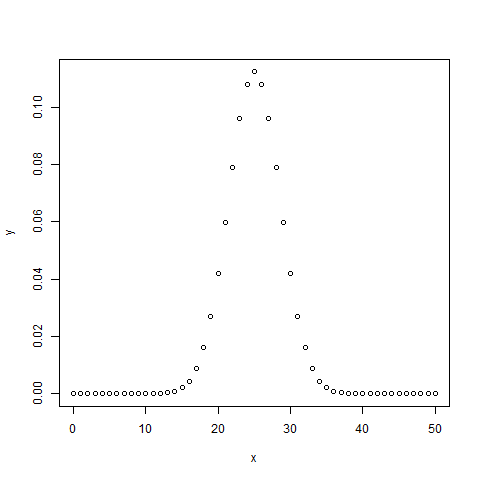R语言 二项分布

R语言有四个内置函数来生成二项分布。 它们描述如下。

dbinom(x, size, prob)
pbinom(x, size, prob)
qbinom(p, size, prob)
rbinom(n, size, prob)

• x是数字的向量。
• p是概率向量。
• n是观察的数量。
• size是试验的数量。
• prob是每个试验成功的概率。

dbinom（）

# Create a sample of 50 numbers which are incremented by 1.
x <- seq(0,50,by = 1)

# Create the binomial distribution.
y <- dbinom(x,50,0.5)

# Give the chart file a name.
png(file = "dbinom.png")

# Plot the graph for this sample.
plot(x,y)

# Save the file.
dev.off()pbinom（）

# Probability of getting 26 or less heads from a 51 tosses of a coin.
x <- pbinom(26,51,0.5)

print(x)

 0.610116

qbinom（）

# How many heads will have a probability of 0.25 will come out when a coin is tossed 51 times.
x <- qbinom(0.25,51,1/2)

print(x)

 23

rbinom（）

# Find 8 random values from a sample of 150 with probability of 0.4.
x <- rbinom(8,150,.4)

print(x)

 58 61 59 66 55 60 61 67